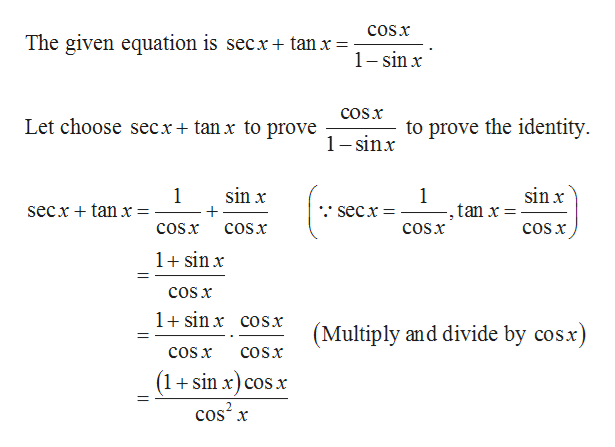Question
18 views

proof trigonometry identities

sec(x)+tan(x)=cos(x)/(1-sin(x))

check_circle

Step 1help_outlineImage TranscriptioncloseCOSx The given equation is secx+ tan x= 1 sin x cOSx Let choose secx+ tan x to prove to prove the identity 1 -sinx sin x sin x 1 secx tan x = 1 tan x = secx COSx coSx COSx cOSx 1sinx cos x 1 sinx coSx (Multiply and divide by cosx) COs x COSX (1+sin x)cos x cos2 x fullscreen

### Want to see the full answer?

See Solution

#### Want to see this answer and more?

Solutions are written by subject experts who are available 24/7. Questions are typically answered within 1 hour.*

See Solution
*Response times may vary by subject and question.
Tagged in

### Other